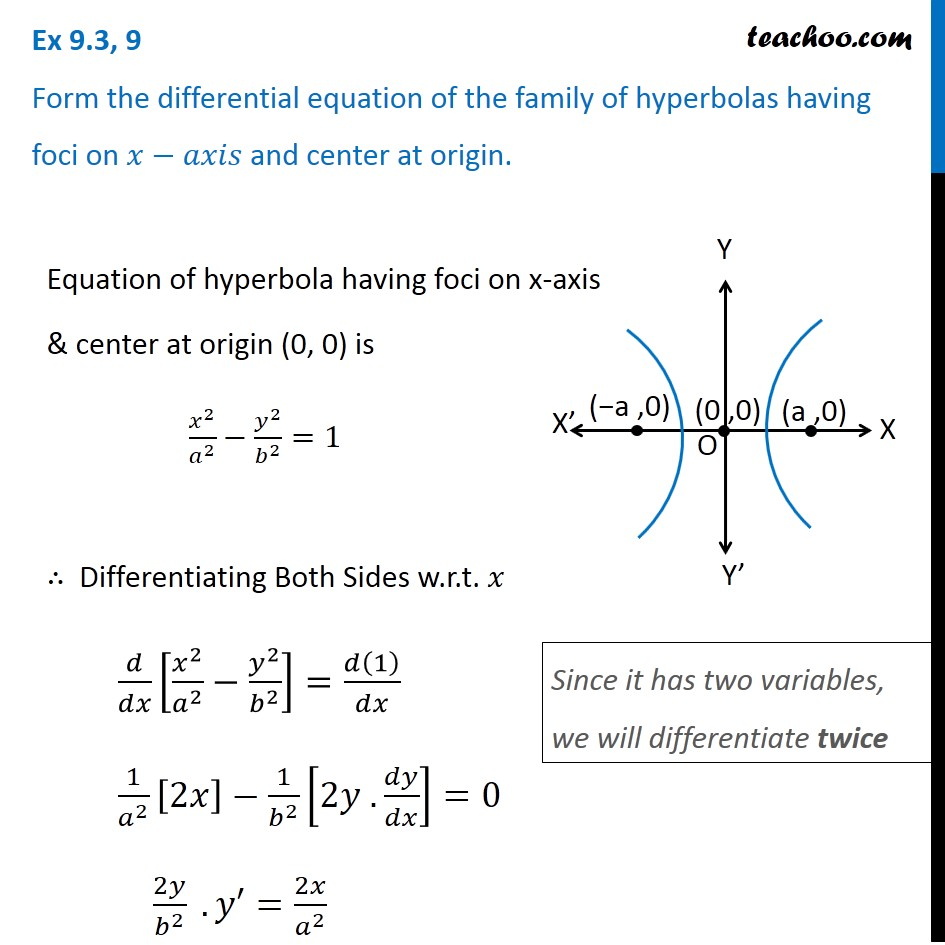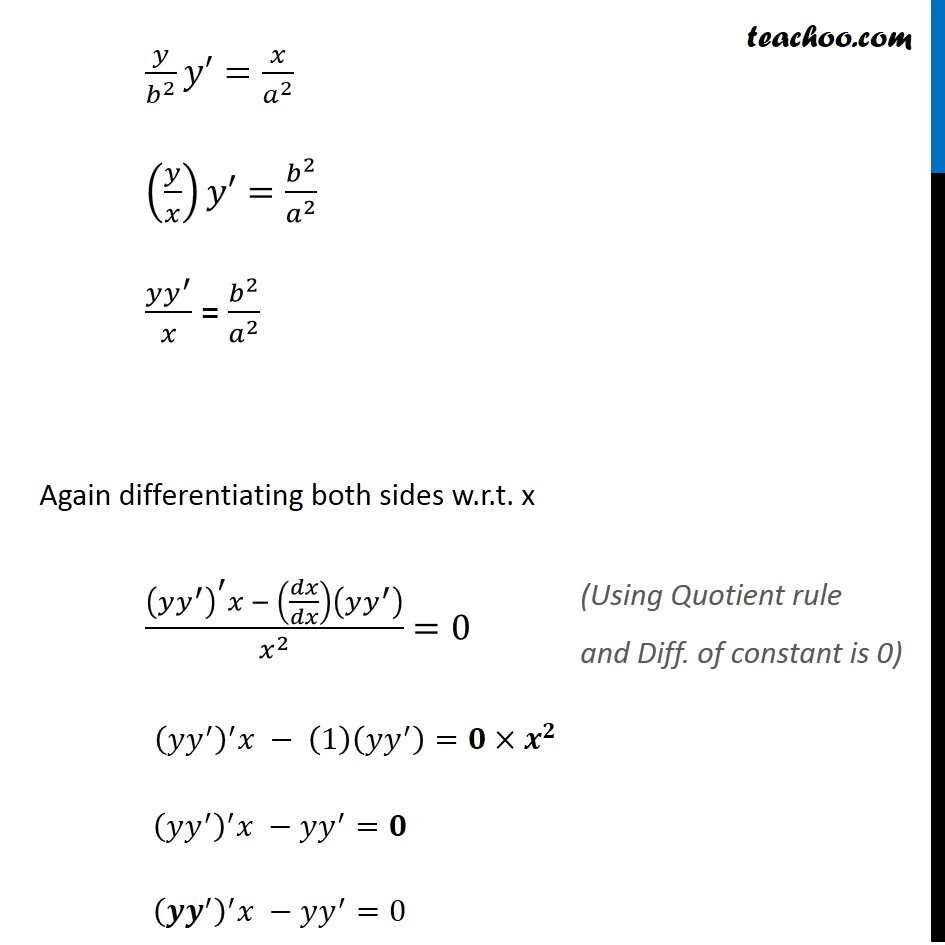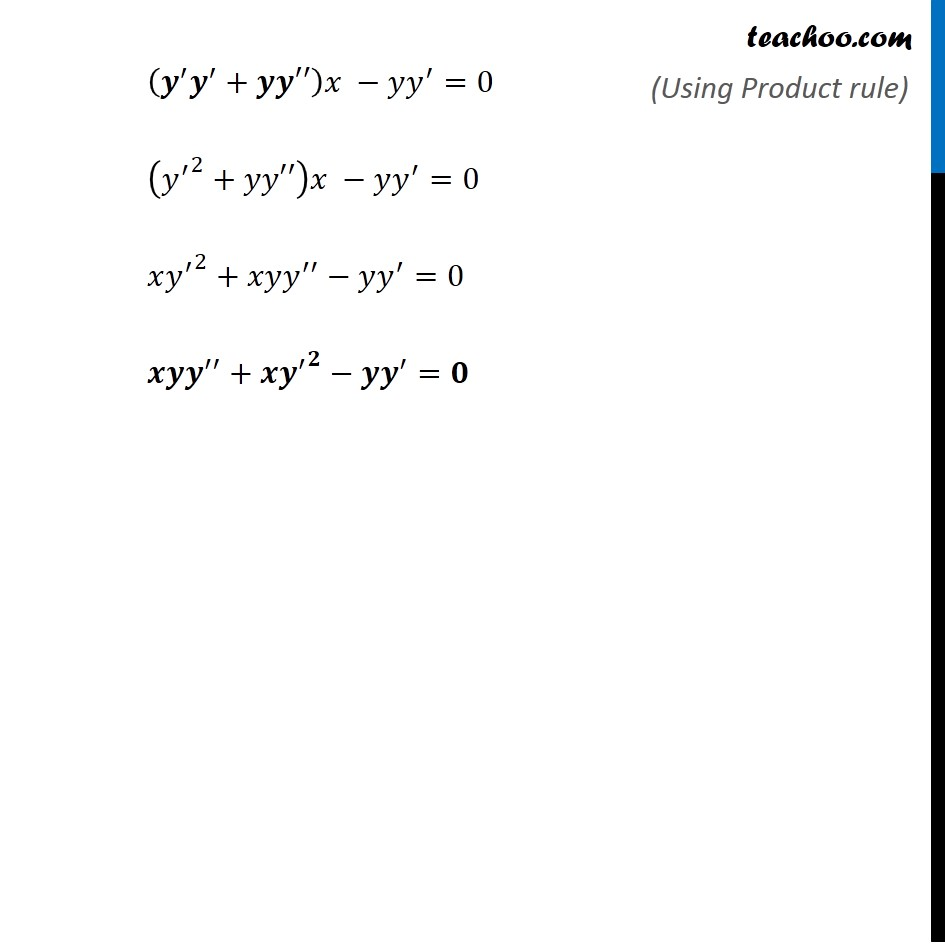Forming Differential equations

Chapter 9 Class 12 Differential Equations
Serial order wiseLearn in your speed, with individual attention - Teachoo Maths 1-on-1 Class

### Transcript

Question 9 Form the differential equation of the family of hyperbolas having foci on 𝑥−𝑎𝑥𝑖𝑠 and center at origin. Equation of hyperbola having foci on x-axis & center at origin (0, 0) is 𝑥^2/𝑎^2 −𝑦^2/𝑏^2 =1 ∴ Differentiating Both Sides w.r.t. 𝑥 𝑑/𝑑𝑥 [𝑥^2/𝑎^2 −𝑦^2/𝑏^2 ]=𝑑(1)/𝑑𝑥 1/𝑎^2 [2𝑥]−1/𝑏^2 [2𝑦 . 𝑑𝑦/𝑑𝑥]=0 2𝑦/𝑏^2 . 𝑦′=2𝑥/𝑎^2 Since it has two variables, we will differentiate twice 𝑦/𝑏^2 𝑦′=𝑥/𝑎^2 (𝑦/𝑥)𝑦′=𝑏^2/𝑎^2 (𝑦𝑦^′)/𝑥 = 𝑏^2/𝑎^2 Again differentiating both sides w.r.t. x ((𝑦𝑦^′ )^′ 𝑥 − (𝑑𝑥/𝑑𝑥)(𝑦𝑦^′ ))/𝑥^2 =0 (𝑦𝑦^′ )^′ 𝑥 − (1)(𝑦𝑦^′ )=𝟎×𝒙^𝟐 (𝑦𝑦^′ )^′ 𝑥 −𝑦𝑦^′=𝟎 (𝒚𝒚^′ )^′ 𝑥 −𝑦𝑦^′=0 (Using Quotient rule and Diff. of constant is 0) (𝒚^′ 𝒚^′+𝒚𝒚′′)𝑥 −𝑦𝑦^′=0 (〖𝑦^′〗^2+𝑦𝑦′′)𝑥 −𝑦𝑦^′=0 𝑥〖𝑦^′〗^2+𝑥𝑦𝑦^′′−𝑦𝑦^′=0 𝒙𝒚𝒚^′′+𝒙〖𝒚^′〗^𝟐−𝒚𝒚^′=𝟎 (Using Product rule)